# 配对交易

```import numpy as np
import pandas as pd```

# 平稳/非平稳是均值和是标准差。标准差的平方，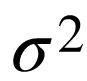，是方差。经验法则规定 66% 的数据应该介于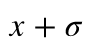和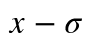，这意味着该函数`normal` 更有可能返回靠近均值的样本，而不是那些远离均值的样本。

```    mu
sigma
return normal(mu, sigma )```

```# 设置参数和数据点数
T = 100

Series(index=range(T))

# 现在参数依赖于时间
# 具体来说，序列的均值随时间变化
B\[t\] = genedata

plt.subplots```

# 为什么平稳性很重要

### 随时关注您喜欢的主题

```np.mean

plt.figure
plt.plot
plt.hlines```

# 为了测试平稳性，我们需要测试一个叫做_单位根的_东西 。自回归单位根检验基于以下假设检验：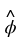是最小二乘估计和 SE(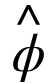) 是通常的标准误差估计。该测试是单侧左尾测试。如果 {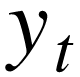} 是平稳的，那么可以证明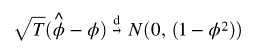或者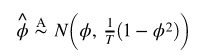并且是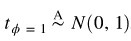，然而，在非平稳性原假设下，上述结果给出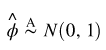以下函数将允许我们使用 Augmented Dickey Fuller (ADF) 检验来检查平稳性。

```defty_test(X, cutoff=0.01):
# 我们必须观察显着的 p 值看该序列是平稳的

# 协整

```# 生成每日收益

np.random.normal

# 总结

plot

np.random.normal
Y = X + 6 + 噪音

plt.show()```
```(Y - X).plot # 绘制点差
plt.axhline# 添加均值
plt.xlabel
plt.xlim```

# 协整检验

1. 检验每个分量系列的单位根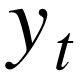单独使用单变量单位根检验，例如 ADF、PP 检验。
2. 如果单位根不能被拒绝，那么下一步就是检验分量之间的协整关系，即检验是否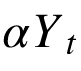是 I(0)。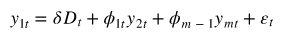是确定性项。假设检验如下：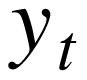与 归一化的协整向量协整

```coint
print(pvalue)

# 低p值意味着高协整！```

# 相关与协整

```Xruns = np.random.normal
yrurs = np.random.normal

pd.concat
plt.xlim```

```Y2 = pd.Series

plt.figure
Y2.plot()

# 相关性几乎为零

prinr(pvle))```

```import fix_yaance as yf
yf.pdrde```

# 交易中的数据科学

```def fitirs(data):
n = data.shape
srmaix = np.zeros
pvl_mrix = np.ones
keys = dta.keys
for i in range(n):
for j in range:

reut = coint
sr = ret\[0\]
paue = rsult\[1\]
soeix\[i, j\] = score
pu_trix\[i, j\] = palue
if palue < 0.05:
pairs.append
return soe_mati, prs```

```start = datetime.datetime
end = datetime.datetime

df = pdr(tcrs, strt, nd)\['Close'\]
df.tail()```
```# 热图显示每对之间的协整检验的 p 值股票。 只显示热图上对角线上的值

seaborn.heatmap```

```coit
pvalue```

# 计算价差

```results.params

sed = S2 - b * S1
sedplot
plt.axhline
plt.xlim
plt.legend```

```rio
rao.plot
plt.axhline
plt.xlim
plt.legend```

z 分数是数据点与平均值的标准差数。更重要的是，高于或低于总体平均值的标准差的数量来自原始分数。z-score 的计算方法如下：

```def zscr:
return (sres - ees.mean) / np.std

zscr.plot
plt.axhline
plt.axhline
plt.axhline
plt.xlim
plt.show```

# 训练测试拆分

```ratios = df\['ADBE'\] / df\['MSFT'\]
print(len(ratios) * .70 )```
```tran = ratos\[:881\]
tet = rats\[881:\]```

# 特征工程

• 60 天移动平均线
• 5 天移动平均线
• 60 天标准差
• z 分数
```train.rolg
zcoe\_5 = (ra\_ag5 - rasag60)/
plt.figure
plt.plot
plt.legend
plt.ylabel
plt.show```
```plt.figure
z5.plot()
plt.xlim
plt.axhline
plt.legend
plt.show```

# 创建模型

• 每当 z-score 低于 -1 时， 买入(1)，这意味着我们预计比率会增加。
• 每当 z 得分高于 1 时，卖出（-1），这意味着我们预计比率会下降。

# 训练优化

```train.plot()
sell
sell\[z5<1\] = 0
sell\[160:\].plot```
```plt.figure

# 当您买入比率时，您买入股票 S1 并卖出 S2

# 当您卖出比率时，您卖出股票 S1 并买入 S2

sell\[sll!=0\] = S1\[sll!=0\]

BuR\[60:\].plot
selR\[60:\].plot```

```# 使用简单的 strydef 进行交易：

# 如果窗口长度为0，算法没有意义，退出

# 计算滚动平均值和滚动标准差
比率 = S1/S2
a1 = rais.rolng
zscoe = (ma1 - ma2)/std

# 模拟交易

# 对于范围内的 i(len(ratios))：
# 如果 z-score > 1，则卖空

mey += S1\[i\] - S2\[i\] * rts\[i\]

cutS2 += raos\[i\]

# 如果 z-score < -1，则买入多头
ef zoe\[i\] > 1:
mey  -= S1\[i\] - S2\[i\] * rtos\[i\]

# 如果 z-score 介于 -.5 和 .5 之间，则清除
elif abs(zcre\[i\]) < 0.75：
mey  += S1\[i\] * ctS + S2\[i\] * oS2```
`trad`

# 改进的领域和进一步的步骤

1. 使用更多的证券和更多样化的时间范围

2. 处理过拟合

3. 调整交易信号

4. 更高级的方法

Kaizong Ye拓端研究室（TRL）的研究员。

​非常感谢您阅读本文，如需帮助请联系我们！QQ在线咨询

15121130882

0571-63341498

## 关注有关新文章的微信公众号

This will close in 0 seconds